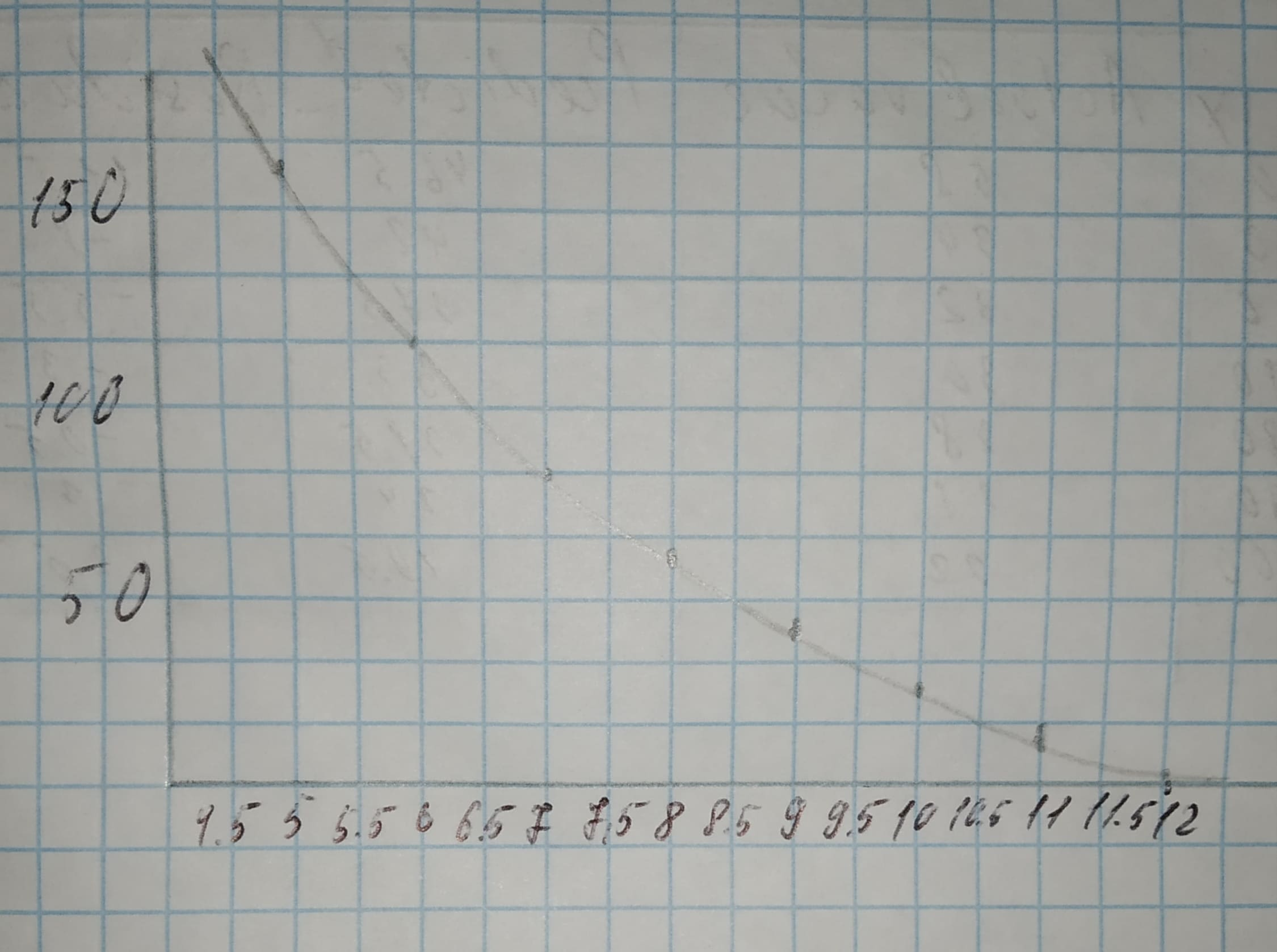Rui Baldwin

2020-11-16

Perform the inverse transformation to express light intensity as an exponential function of depth in the lake.
Display a scatterplot of the original data with exponential model superimposed. Is your exponential function a satisfactory model for the data?yagombyeR

Step 1
Equation found in exercise 5d (where $\stackrel{^}{y}$ is the natural logarithm of the light intensity):
$\left[\stackrel{^}{y}=6.7891-0.330x\right]$
Take the exponential of both sides:
$\left[\stackrel{^}{y}={e}^{6.7891-0.330x}={e}^{6.7891}{e}^{-0.330x}\right]$
Step 2
The Depth is on the vertical axis and the light intensity is on the horizontal axis.Step 3
The model is an satisfactory model, because it seems to pass through every single data point.
Result:
Yes.

Do you have a similar question?